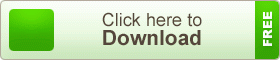# SSC MCQ Question Ans. Trigonometric Ratio

SSC MCQ Question Ans. Trigonometric Ratio. In our day to day life we make use of triangles,  and in particular, right-angled triangles. Many different examples from our surroundings can be drawn where right triangles can be imagined to be formed. In ancient times, with the help of geometry men learned the technique of determining the width of a river by standing on its bank. Without climbing the tree they knew how to measure the height of the tree accurately by comparing its shadow with that of a stick. In all the situations given above, the distances or heights can be found by using some mathematical technique which comes under a special branch of mathematics called Trigonometry.

## SSC MCQ Question Ans. Trigonometric Ratio###teachingbd24.com is such a website where you would get all kinds of necessary information regarding educational notes, suggestions and questions’ patterns of school, college, and madrasahs. Particularly you will get here special notes of physics that will be immensely useful to both students and teachers. The builder of the website is Mr. Md. Shah Jamal Who has been serving for 30 years as an Asst. Professor of BAF Shaheen College. He expects that this website will meet up all the needs of Bengali version learners /students. He has requested concerned both students and teachers to spread this website home and abroad.The word ‘Trigonometry’ is derived from the SSC MCQ Question Ans. Trigonometric RatioGreek words ‘tri’ (means three), ‘gon’ (means edge) and `matron’  (means measure).  In fact, trigonometry is the study of the relationship between the sides and angles of a triangle. There is evidence of using the Trigonometry in Egyptian and Babylon civilization. It is believed that the Egyptians made its extensive use in land survey and engineering works. Early astrologer used it to determine the distances from the Earth to the farthest planets and stars. At present trigonometry is in use in all branches of mathematics.  There are wide usages of trigonometry for the solution of triangle related problems and in navigation etc. Nowadays trigonometry is in wide use in Astronomy and Calculus.